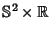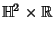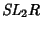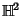## Thurston's Geometrization Conjecture

Thurston's conjecture has to do with geometric structures on 3-D Manifolds. Before stating Thurston's conjecture, some background information is useful. 3-dimensional Manifolds possess what is known as a standard 2-level Decomposition. First, there is the Connected Sum Decomposition, which says that every Compact 3-Manifold is the Connected Sum of a unique collection of Prime 3-Manifolds.

The second Decomposition is the Jaco-Shalen-Johannson Torus Decomposition, which states that irreducible orientable Compact 3-Manifolds have a canonical (up to Isotopy) minimal collection of disjointly Embedded incompressible Tori such that each component of the 3-Manifold removed by the Tori is either atoroidal'' or Seifert-fibered.''

Thurston's conjecture is that, after you split a 3-Manifold into its Connected Sum and then Jaco-Shalen-Johannson Torus Decomposition, the remaining components each admit exactly one of the following geometries:

1. Euclidean Geometry,

2. Hyperbolic Geometry,

3. Spherical Geometry,

4. the Geometry of,

5. the Geometry of,

6. the Geometry of,

7. Nil Geometry, or

8. Sol Geometry.
Here,is the 2-Sphere andis the Hyperbolic Plane. If Thurston's conjecture is true, the truth of the Poincaré Conjecture immediately follows.

See also Connected Sum Decomposition, Euclidean Geometry, Hyperbolic Geometry, Jaco-Shalen-Johannson Torus Decomposition, Nil Geometry, Poincaré Conjecture, Sol Geometry, Spherical Geometry